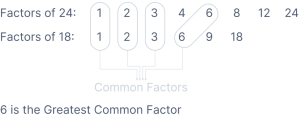# Factor Calculator

Factorization in mathematics is dividing a large number into smaller ones that, when multiplied together, give you the original number. The Mathematics Master Factor Calculator is used to find the positive or negative number's factors and factor pairs.

## What is a Factor?

The integers are multiplied together to produce factors in multiplication. For instance, $$7 × 2 = 14$$. In this case, the factors of 14 are 7 and 2. Therefore, the components of 14 would also include 1, 2, 7, and 14.

The original numbers influence the product number. For example, a and b are factors of c if $$a × b = c$$.

## Basic Factorisation Formula

$$a^2 – b^2 = (a – b)(a + b)$$

$$(a + b)^2 = a^2 + 2ab + b^2$$

$$(a – b)^2 = a^2 – 2ab + b^2$$

$$a^3 – b^3 = (a – b)(a^2 + a b + b^2)$$

$$a^3 + b^3 = (a + b)(a^2 – ab + b^2)$$

$$(a + b + c)^2 = a^2 + b^2 + c^2 + 2ab + 2bc + 2ca$$

$$(a – b – c)^2 = a^2 + b^2 + c^2 – 2ab + 2bc – 2ca$$

## Examples

Example 1: Let’s factor $$x^2 + 8x + 16$$

The middle number is 8 and the last number is 16.

For factoring them we want something like, (x + _)(x + _)

We need two numbers that add them together to get 8 and multiply together to get 16.

We will try 4 and 4:

$$4 + 4 = 8$$

$$4 × 4 = 16$$

Answer: $$(x + 4)(x + 4)$$

Example 2: Factorize $$x^3 − x^2 − 9x + 9$$

First, we will factor the first two and second two separately:

$$x^2(x − 1) − 9(x − 1)$$

We see that (x-1) is on both, so let us use that:

$$(x^2 − 9)(x − 1)$$

And $$x^2 − 9$$ can be simplified as: $$(x – 3) (x + 3)$$

Answer: $$(x − 3)(x + 3)(x − 1)$$

## FAQs

What is the greatest common factor?

Greatest Common Factor of 24 and 18

1. Identify every factor of each number.
2. Mark all of the common factors.
3. Select the Greatest factorsAlternatively, you can use an online greatest common factor calculator.

Find the greatest common factor of $$9x^7 + 6x^4 − 3x^3$$.

Given, $$9x^7 + 6x^4 − 3x^3$$Now factoring in the given expression, we get;

$$= 3x^3 ( 3x^4 + 2x − 1 )$$

Therefore, the greatest common factor is $$3x^3$$.

What is a scale factor calculator?

For scale conversion, i.e., to scale up or down a measurement, you need a standard scale factor with which you can multiply or divide all measures. An online scale factor calculator is used for scale conversions.